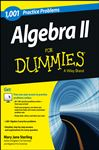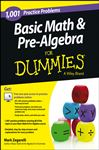AlgebraHome   Algebra   Geometry   Arithmetic   Trigonometry   Probability/Statistics   Calculus   Mechanics GED   SAT   ACT   College Mathematics   GRE   School Supplies   About Us

Algebra

 Algebra Calculations - Downloads Only (for Windows) - \$14.99 Application will calculate for you while giving an understanding of each topic. Radians, Arcs and Sectors, Reciprocals, Percent, Factorials Permutations, Combinations, Simple and Compound Interests Order of Operations, Ratio and Proportions Co-ordinates and the Straight Line, Length of a Straight Line Mid-point of a Straight Line, Gradient of a Straight Line, Equation of a Straight Line Graphs of a Straight Line, Logarithms, Pythagorean Theorem Geometric Progression, Arithmetic Progression, Harmonic Progression, Arithmetic Mean Arithmetic Sequence, Common Difference, Sum of Arithmetic Series Geometric Sequence, Common Ratio, Sum of Geometric Series Quadratic Solutions, Exponents (Indices), Negative Exponents Fractional Exponents, Laws of Exponents, Natural Logarithms, Exponential Function Common Logarithms, Antilogarithms, Summation Notation Inequalities, Simple Equations Instructions will be sent to you through the email provided within 24 hrs. of purchase.Electronic Textbooks TDownloads - Preview, Get, Practice!Paperback Textbooks (New and Used)Examples

 Inequalities: Solve 3y + 4 > 2(y + 3) + y     3y + 4 - 4 > 2y + 6 + y - 4  (Subtracting 4 from each side.)         3y + 0 > 2y + y + 6 - 4  (Grouping like terms.)               3y > 3y + 2                  3y - 3y > 3y -3y + 2  (Subtracting 3y from both sides.)                0 > 0 + 2 Answer: Since y is eliminated, there are no             values to display. Proportions: Solve for x,  (2x + 8):(x + 2) = (2x + 5):(x + 1)     By Proportions  (2x+8)  =  (2x+5)                            (x+2)        (x+1)       (2x+8)(x+1) = (2x+5)(x+2)  (Finding the Cross Products)  2x2 + 8x + 2x + 8 = 2x2 +5x + 4x + 10  (Expanding)        2x2 + 10x + 8 = 2x2 + 9x + 10 2x2 - 2x2 + 10x - 9x = 10 - 8    (Rearranging)                           x  = 2

 Permutations: A permutation of a set of objects is an ordered arrangement of all the objects. How many permutations are there of a set of 4 objects, say {A, B, C, D}? Answer: Number of permutations is 4 X 3 X 2 X 1 = 24

 Functions: Given the function f(x) = 2x� - 5x + 3.  Find the values of x and draw a graph of the function.                   Let y = f(x) = 2x� - 5x + 3                      If y = f(x)               Then y = 2x� - 5x + 3                         y = (x - 1)(2x - 3) by factorization                When y = 0, x - 1 = 0 and 2x - 3 = 0                    When x - 1 = 0, x = 1                       When 2x - 3 = 0, x = 3/2 = 1�Values of x are 1 and 1�The solutions are the intercepts of the graph on the x   axis, 1 and 1�.for Math Practice,   SAT  ACT

 More School Supplies and other useful items *  * *